Ch 8. Strain Analysis Multimedia Engineering Mechanics PlaneStrain Mohr's Circlefor Strain StrainGages
 Chapter 1. Stress/Strain 2. Torsion 3. Beam Shr/Moment 4. Beam Stresses 5. Beam Deflections 6. Beam-Advanced 7. Stress Analysis 8. Strain Analysis 9. Columns Appendix Basic Math Units Basic Equations Sections Material Properties Structural Shapes Beam Equations Search eBooks Dynamics Fluids Math Mechanics Statics Thermodynamics Author(s): Kurt Gramoll ©Kurt GramollMECHANICS - THEORY Strain Gage Basics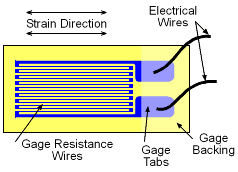Basic Linear Strain Gage It is not possible (currently) to measure stress directly in a structure. However, it is possible to measure strain since it is based on displacement. There are a number of techniques to measure strain but the two more common are extensometers (monitors the distance between two points) and strain gages. Strain gages are constructed from a single wire that is wound back and forth. The gage is attached to the surface of an object with wires in the direction where the strain is to be measured. The electrical resistance in the wires change when they are elongated. Thus, the voltage change in the wires can be collaborated to the change in strain. Most strain gage measurement devices automatically collaborate the voltage change to the strain, so the device output is the actual strain. Strain Rosette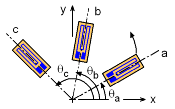Strain Gage Rosette at Arbitrary Angles Since a single gage can only measure the strain in only a single direction, two gages are needed to determine strain in the εx and εy. However, there is no gage that is capable of measuring shear strain. There is a clever solution to finding shear strain. Three gages are attached to the object in any three different angles. Recall, any rotated normal strain is a function of the coordinate strains, εx, εy and γxy, which are unknown in this case. Thus, if three different gages are all rotated, that will give three equations, with three unknowns, εx, εy and γxy. These equations are,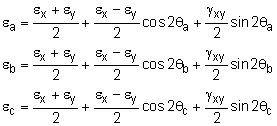Any three gages used together at one location on a stressed object is called a strain rosette. Strain Rosette - 45o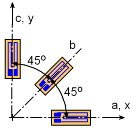Strain Gage Rosette at 45o To increase the accuracy of a strain rosette, large angles are used. A common rosette of three gages is where the gages are separated by 45o, or θa = 0o, or θb = 45o, or θc = 90o. The three equations can then be simplify to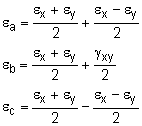Solving for εx, εy and γxy gives,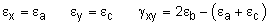Strain Rosette - 60o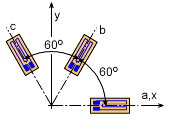Strain Gage Rosette at 60o Similarly, if the angles between the gages are 60o, or θa = 0o, or θb = 60o, or θc = 120o., the unknown strains, for εx, εy and γxy will be,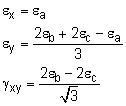Practice Homework and Test problems now available in the 'Eng Mechanics' mobile app
Includes over 400 problems with complete detailed solutions.
Available now at the Google Play Store and Apple App Store.